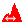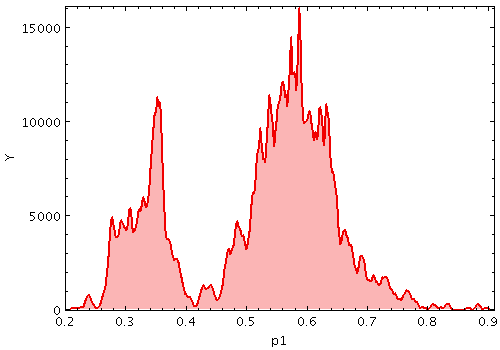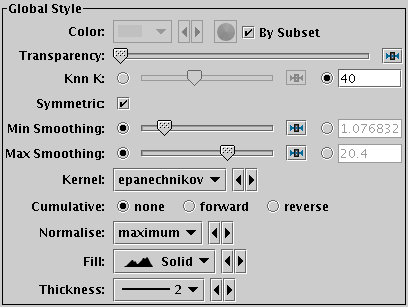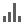Next Previous Up Contents
Next: Densogram Form
Up: Plot Forms
Previous: KDE Form

#### A.4.5.23 KNN Form

The KNN form () plots a discrete Kernel Density Estimate giving a smoothed frequency of data values along the horizontal axis, using an adaptive (K-Nearest-Neighbours) smoothing kernel. This is a generalisation of a histogram in which the bins are always 1 pixel wide, and a variable-bandwidth smoothing kernel is applied to each bin (for a fixed-bandwidth kernel, see KDE).

The K-Nearest-Neighbour figure gives the number of points in each direction to determine the width of the smoothing kernel for smoothing each bin. Upper and lower limits for the kernel width are also supplied; if the upper and lower limits are equal, this is equivalent to a fixed-width kernel.

Note this is not a true Kernel Density Estimate, since, for performance reasons, the smoothing is applied to the (pixel-width) bins rather than to each data sample. The deviation from a true KDE caused by this quantisation will be at the pixel level, hence in most cases not visually apparent.

This form may be used in either the Histogram, Plane or Time plot windows.Example KNN plotKNN form configuration panel

These options always appear in the form configuration panel:

Colour
Selects the basic colour for the dataset.
Transparency
Adjusts the transparency of the bars or line. For bar styles which are already partially transparent, this fades them further.
Knn K
Sets the number of nearest neighbours to count away from a sample point to determine the width of the smoothing kernel at that point. For the symmetric case this is the total of nearest neighbours summed over both directions, and for the asymmetric case it is the number in a single direction. The threshold is actually the weighted total of samples; for unweighted (weight=1) bins that is equivalent to the number of samples.
Symmetric
If checked, the nearest neigbour search is carried out in both directions, and the kernel is symmetric. If unchecked, the nearest neigbour search is carried out separately in the positive and negative directions, and the kernel width is accordingly different in the positive and negative directions.
Min Smoothing
Fixes the minimum size of the smoothing kernel. This functions as a lower limit on the distance that is otherwise determined by searching for the K nearest neighbours at each sample point.

You can either use the slider, or check the radio button on the right and enter the value in data units directly.

Max Smoothing
Fixes the maximum size of the smoothing kernel. This functions as an upper limit on the distance that is otherwise determined by searching for the K nearest neighbours at each sample point.

You can either use the slider, or check the radio button on the right and enter the value in data units directly.

Fill
Determines how the density function is represented. The options are:
• solid: area between level and axis is filled with solid colour
• line: level is marked by a wiggly line
• semi: level is marked by a wiggly line, and area below it is filled with a transparent colour
Thickness
Controls line thickness where applicable. This is only relevant for bar styles that draw a finite thickness line, so has no effect for solid filling.
And these options appear in the form configuration panel for the Plane window, or the Bins control () for the Histogram window:
Kernel
The functional form of the smoothing kernel. The functions listed refer to the unscaled shape; all kernels are normalised to give a total area of unity.

The available options are:

• square: Uniform value: f(x)=1, |x|=0..1
• linear: Triangle: f(x)=1-|x|, |x|=0..1
• epanechnikov: Parabola: f(x)=1-x*x, |x|=0..1
• cos: Cosine: f(x)=cos(x*pi/2), |x|=0..1
• cos2: Cosine squared: f(x)=cos^2(x*pi/2), |x|=0..1
• gauss3: Gaussian truncated at 3.0 sigma: f(x)=exp(-x*x/2), |x|=0..3
• gauss6: Gaussian truncated at 6.0 sigma: f(x)=exp(-x*x/2), |x|=0..6

Cumulative
If set to forward or reverse, the heights are plotted cumulatively; each bin includes the counts from all previous bins in the direction of negative or positive infinity.
Normalise
Defines how, if at all, the bars are normalised. The available options are:
• none: No normalisation is performed.
• area: The total area of histogram bars is normalised to unity. For cumulative plots, this behaves like height.
• unit: Histogram bars are scaled by the inverse of the bin width in data units. For cumulative plots, this behaves like none.
• maximum: The height of the tallest histogram bar is normalised to unity. For cumulative plots, this behaves like height.
• height: The total height of histogram bars is normalised to unity.
When used in the Time plot only, additional options per_second, per_day etc are available corresponding to the frequency over the named time unit.

Next Previous Up Contents
Next: Densogram Form
Up: Plot Forms
Previous: KDE Form

TOPCAT - Tool for OPerations on Catalogues And Tables